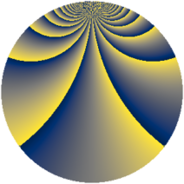# Properties

 Label 1323.2.bsLevel $1323$ Weight $2$ Character orbit 1323.bs Rep. character $\chi_{1323}(26,\cdot)$ Character field $\Q(\zeta_{42})$ Dimension $900$ Sturm bound $336$

# Related objects

## Defining parameters

 Level: $$N$$ $$=$$ $$1323 = 3^{3} \cdot 7^{2}$$ Weight: $$k$$ $$=$$ $$2$$ Character orbit: $$[\chi]$$ $$=$$ 1323.bs (of order $$42$$ and degree $$12$$) Character conductor: $$\operatorname{cond}(\chi)$$ $$=$$ $$147$$ Character field: $$\Q(\zeta_{42})$$ Sturm bound: $$336$$

## Dimensions

The following table gives the dimensions of various subspaces of $$M_{2}(1323, [\chi])$$.

Total New Old
Modular forms 2088 900 1188
Cusp forms 1944 900 1044
Eisenstein series 144 0 144

## Trace form

 $$900q - 76q^{4} + 5q^{7} + O(q^{10})$$ $$900q - 76q^{4} + 5q^{7} - 6q^{10} + 78q^{16} + 3q^{19} - 22q^{22} + 71q^{25} - 30q^{28} + 39q^{31} + 56q^{34} - 69q^{37} + 152q^{40} + 2q^{43} + 10q^{46} + 19q^{49} + 132q^{52} - 336q^{55} - 84q^{58} + 13q^{61} + 152q^{64} - 14q^{67} + 140q^{70} - 51q^{73} + 336q^{76} + 14q^{79} + 52q^{82} - 84q^{85} + 6q^{88} - 254q^{91} - 170q^{94} + O(q^{100})$$

## Decomposition of $$S_{2}^{\mathrm{new}}(1323, [\chi])$$ into newform subspaces

The newforms in this space have not yet been added to the LMFDB.

## Decomposition of $$S_{2}^{\mathrm{old}}(1323, [\chi])$$ into lower level spaces

$$S_{2}^{\mathrm{old}}(1323, [\chi]) \cong$$ $$S_{2}^{\mathrm{new}}(147, [\chi])$$$$^{\oplus 3}$$$$\oplus$$$$S_{2}^{\mathrm{new}}(441, [\chi])$$$$^{\oplus 2}$$